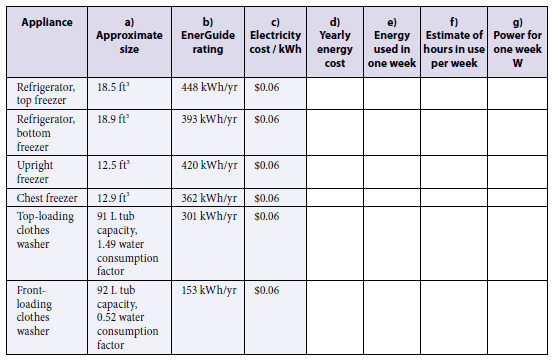# Work, Power, energy: Appliances (refrigerators, freezer etc)

• Evangeline101
In summary, the refrigerators and freezers use 8.62 kWh per week and the washers use 5 hours per week. The upright freezer uses 8.08 kWh per week and the chest freezer uses 6.96 kWh per week. The top-loading clothes washer uses 1.158 kW and the front-loading clothes washer uses 0.58 kW.

## Homework Statement

Fill in the empty columns of the following table:y = ΔE x c
P = ΔE/Δt
ΔE = Y/ Δt

## The Attempt at a Solution

[/B]
Part d) Yearly energy cost:
Use Y=ΔE x c

Refrigerator, top freezer:
Y = (448 kWh/yr) ($0.06) Y =$ 26. 88/yr

Refrigerator, bottom freezer:
Y = (393 kWh/yr) ($0.06) Y =$ 23.58/yr

Upright freezer:
Y = (420 kWh/yr) ($0.06) Y=$ 25.20/yr

Chest freezer:
Y = (362 kWh/yr) ($0.06) Y =$21.72/yr

Y = (301 kWh/yr) ($0.06) Y =$ 18.06 / yr

Y =(153 kWh/yr) ($0.06) Y =$ 9.18/yr

Part e) Energy used in one week:
Use ΔE = Y/Δt

Refrigerator, top freezer:
ΔE = 448 kWh /yr / 52 weeks
= 8.62 kWh/week

Refrigerator, bottom freezer:
ΔE = 393 kWh/yr / 52 weeks
= 7.56 kWh/week

Upright freezer:
ΔE = 420 kWh/yr / 52 weeks
= 8.08 kWh/week

Chest freezer:
ΔE = 362 kWh/yr / 52 weeks
= 6.96 kWh/ week

ΔE = 301 kWh/yr / 52 weeks
= 5.79 kWh/ week

ΔE = 153 kWh/yr / 52 weeks
= 2.9 kWh/week

Part f) Estimate of hours in use per week:

Okay, so for all the refrigerators and freezers, I estimated the hours of use to be 168 hours, since I assumed these appliances are being used all day for the entire week. ( I am not sure if that is correct)

For the washers, I estimated the hours per week to be 5 hours.

Can someone please tell me if my estimate is correct, or do I need to put a different amount of hours for each appliance?

Here is part g) using my estimate of hours per week from part f)
Use P = ΔE/ Δt

Refrigerator, top freezer:
P = 8.62 kWh/ week / 168 hrs/week
= 0.05 kW or 50 W

Refrigerator, bottom freezer:
P = 7.56 kWh /week /168 hrs/week
= 0.045 kW or 45 W

Upright freezer:
P = 8.08 kWh/week / 168 hrs/week
= 0.048 kW or 48 W

Chest freezer:
P = 6.69 kWh/week / 168 hrs/week
= 0.041 kW or 41 W

P = 5.79 kWh /week / 5 hrs/week
= 1.158 kW or 1158 W

P = 2.9 kWh /week / 5 hrs/ week
= 0.58 kW or 580 W

Is my process and answer correct?

Any help would be appreciatedEvangeline101 said:
Okay, so for all the refrigerators and freezers, I estimated the hours of use to be 168 hours, since I assumed these appliances are being used all day for the entire week. ( I am not sure if that is correct)

For the washers, I estimated the hours per week to be 5 hours.
Should be fine if you have to guess values.

The approach is fine everywhere, didn't check all the numbers, computers can do that better.

Real freezers tend to switch between cooling and staying passive frequently, but the duty cycle will depend on the specific product.

If I changed the hours of use per week to between 80 and 100 hours, would that make sense?

Like this:

Top freezer: 95 hrs
bottom freezer: 85 hrs
upright freezer: 90hrs
chest freezer: 80 hrs

I am estimating the hours, so I can choose any values right?

I'm not sure if that is a better answer.

mfb said:
I'm not sure if that is a better answer.

Okay, so should I just use my original estimated hours?

I would use those.

mfb said:
I would use those.

Okay, but does it make sense that the power for the freezers are so low while the power for the washers are high?

Why do you think this would be an issue?

mfb said:
Why do you think this would be an issue?

Well, I just thought that since the freezers are being used more hours per week the power would be higher.. but I guess that is not what they are asking of me in this question.

Anyway, thanks for the help!